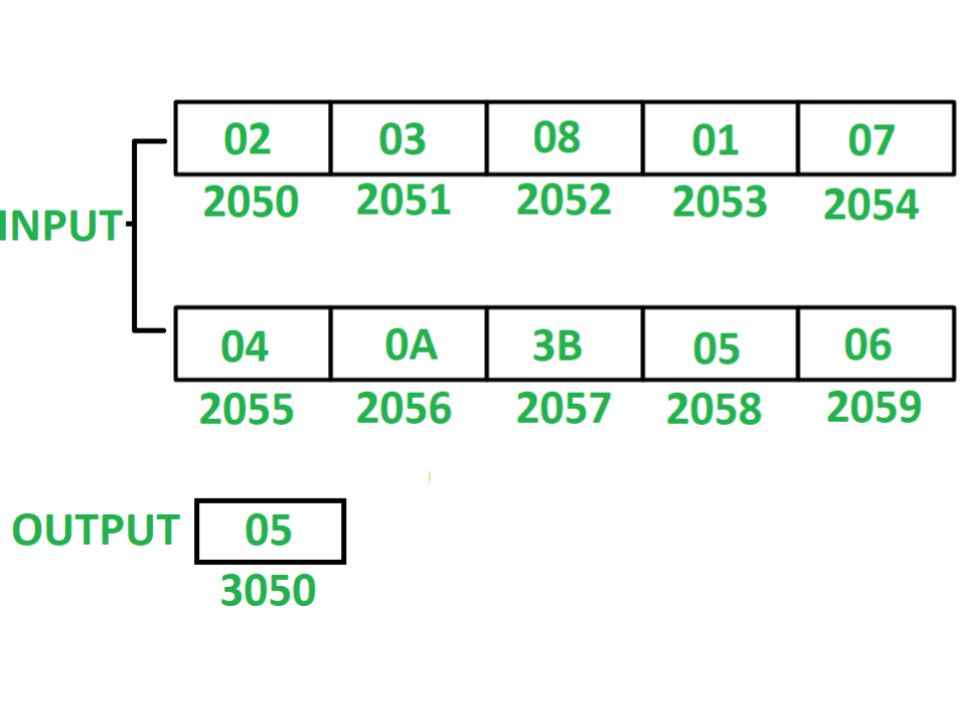8085 program to count total even numbers in series of 10 numbers

• Last Updated : 01 Jun, 2018

Program – Write an assembly language program in 8085 microprocessor to count even numbers in series of 10 numbers.

Example –Assumption – Ten 8-bit numbers are stored from starting memory location 2050. Value of count is stored at memory location 3050.

Algorithm –

1. Initialize register H with 20 and register L with 4F so that indirect memory points to memory location 204F.
2. Initialize register C with 00 and register D with 0A.
3. Increment indirect memory by 1.
4. Store value of M in accumulator A.
5. Check whether the content in A is even or odd by performing AND operation of A with 01.
6. If content of A is 00 after AND operation then number scanned was even, If so then increment C by 01 else if content of A is 01 after AND operation then number scanned was odd, If so then decrements D by 01.
7. Check if zero flag is not set i.e. ZF = 0 then jump to step 3 otherwise store value of C at memory location 3050.

Program –

2000LXI H 204FH <- 20, L <- 4F
2003MVI C, 00C <- 00
2007INX HM <- M + 01
2008MOV A, MA <- M
2009ANI 01A <- A (AND) 01
200BJNZ 200FJump if ZF = 0
200EINR CC <- C + 01
200FDCR DD <- D – 01
2010JNZ 2007Jump if ZF = 0
2013MOV A, CA <- C
2014STA 3050M <- A
2017HLTEND

Explanation – Registers A, B, C, D, H, L are used for general purpose.

1. LXI H 204F: assign 20 to H and 4F to L.
2. MVI C, 00: assign 00 to C.
3. MVI D, 0A: assign 0A to D.
4. INX H: increment indirect memory location M by 01.
5. MOV A, M: move content of M to A.
6. ANI 01: perform AND operation of A with 01 and store the result in A.
7. JNZ 200F: jump if ZF = 0 to memory location 200F.
8. INR C: increment C by 01.
9. DCR D: decrements D by 01.
10. JNZ 2007: jump if ZF = 0 to memory location 2007.
11. MOV A, C: moves the content of C to A.
12. STA 3050: store the content of A to memory location 3050.
13. HLT: stops executing the program and halts any further execution.
My Personal Notes arrow_drop_up